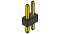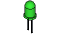CIRC02
 .:8 LED Fun:..:Multiple LEDs:.(ARDX) .:Arduino Expermentation Kit:. (ARDX)

What We're Doing
We have caused one LED to blink, now it's time to up the stakes. Lets connect eight. We'll also have an opportunity to stretch the Arduino a bit by creating various lighting sequences. This circuit is also a nice setup to experiment with writing your own programs and getting a feel for how the Arduino works.

Along with controlling the LEDs we start looking into a few simple programming methods to keep your programs small.

for() loops - used when you want to run a piece of code several times.
arrays[] - used to make managing variables easier (it's a group of variables).

The Circuit
The PartsCIRC-02Breadboard Sheetx12 Pin Headerx45mm Green LEDx8Wire560 Ohm ResistorGreen-Blue-Brownx8SchematicResources
http://ardx.org/BBLS02

.:view:.
assembly video
http://ardx.org/VIDE02Code (no need to type everything in just)
(and then copy the text and paste it into an empty Arduino Sketch)

/*     ---------------------------------------------------------
*     |  Arduino Experimentation Kit Example Code             |
*     |  CIRC-02 .: 8 LED Fun :. (Multiple LEDs)   |
*     ---------------------------------------------------------
*
*  A few Simple LED animations
*
* For more information on this circuit http://tinyurl.com/d2hrud
*
*/

//LED Pin Variables
int ledPins[] = {2,3,4,5,6,7,8,9}; //An array to hold the pin each LED is connected to
//i.e. LED #0 is connected to pin 2, LED #1, 3 and so on
//to address an array use ledPins this would equal 2
//and ledPins would equal 9

/*
* setup() - this function runs once when you turn your Arduino on
* We the three control pins to outputs
*/
void setup()
{

//Set each pin connected to an LED to output mode (pulling high (on) or low (off)
for(int i = 0; i < 8; i++){         //this is a loop and will repeat eight times
pinMode(ledPins[i],OUTPUT); //we use this to set each LED pin to output
}                                   //the code this replaces is below

/* (commented code will not run)
* these are the lines replaced by the for loop above they do exactly the
* same thing the one above just uses less typing
pinMode(ledPins,OUTPUT);
pinMode(ledPins,OUTPUT);
pinMode(ledPins,OUTPUT);
pinMode(ledPins,OUTPUT);
pinMode(ledPins,OUTPUT);
pinMode(ledPins,OUTPUT);
pinMode(ledPins,OUTPUT);
pinMode(ledPins,OUTPUT);
(end of commented code)*/
}

/*
* loop() - this function will start after setup finishes and then repeat
* we call a function called oneAfterAnother(). if you would like a different behaviour
* uncomment (delete the two slashes) one of the other lines
*/
void loop()                     // run over and over again
{
oneAfterAnotherNoLoop();   //this will turn on each LED one by one then turn each off
//oneAfterAnotherLoop();   //does the same as oneAfterAnotherNoLoop but with
//much less typing
//oneOnAtATime();          //this will turn one LED on then turn the next one
//on turning the
//former off (one LED will look like it is scrolling
//along the line
//inAndOut();              //lights the two middle LEDs then moves them out then back
//in again
}

/*
* oneAfterAnotherNoLoop() - Will light one LED then delay for delayTime then light
* the next LED until all LEDs are on it will then turn them off one after another
*
* this does it without using a loop which makes for a lot of typing.
* oneOnAtATimeLoop() does exactly the same thing with less typing
*/
void oneAfterAnotherNoLoop(){
int delayTime = 100; //the time (in milliseconds) to pause between LEDs
//make smaller for quicker switching and larger for slower
digitalWrite(ledPins, HIGH);  //Turns on LED #0 (connected to pin 2 )
delay(delayTime);                //waits delayTime milliseconds
digitalWrite(ledPins, HIGH);  //Turns on LED #1 (connected to pin 3 )
delay(delayTime);                //waits delayTime milliseconds
digitalWrite(ledPins, HIGH);  //Turns on LED #2 (connected to pin 4 )
delay(delayTime);                //waits delayTime milliseconds
digitalWrite(ledPins, HIGH);  //Turns on LED #3 (connected to pin 5 )
delay(delayTime);                //waits delayTime milliseconds
digitalWrite(ledPins, HIGH);  //Turns on LED #4 (connected to pin 6 )
delay(delayTime);                //waits delayTime milliseconds
digitalWrite(ledPins, HIGH);  //Turns on LED #5 (connected to pin 7 )
delay(delayTime);                //waits delayTime milliseconds
digitalWrite(ledPins, HIGH);  //Turns on LED #6 (connected to pin 8 )
delay(delayTime);                //waits delayTime milliseconds
digitalWrite(ledPins, HIGH);  //Turns on LED #7 (connected to pin 9 )
delay(delayTime);                //waits delayTime milliseconds

//Turns Each LED Off
digitalWrite(ledPins, LOW);  //Turns on LED #0 (connected to pin 2 )
delay(delayTime);                //waits delayTime milliseconds
digitalWrite(ledPins, LOW);  //Turns on LED #1 (connected to pin 3 )
delay(delayTime);                //waits delayTime milliseconds
digitalWrite(ledPins, LOW);  //Turns on LED #2 (connected to pin 4 )
delay(delayTime);                //waits delayTime milliseconds
digitalWrite(ledPins, LOW);  //Turns on LED #3 (connected to pin 5 )
delay(delayTime);                //waits delayTime milliseconds
digitalWrite(ledPins, LOW);  //Turns on LED #4 (connected to pin 6 )
delay(delayTime);                //waits delayTime milliseconds
digitalWrite(ledPins, LOW);  //Turns on LED #5 (connected to pin 7 )
delay(delayTime);                //waits delayTime milliseconds
digitalWrite(ledPins, LOW);  //Turns on LED #6 (connected to pin 8 )
delay(delayTime);                //waits delayTime milliseconds
digitalWrite(ledPins, LOW);  //Turns on LED #7 (connected to pin 9 )
delay(delayTime);                //waits delayTime milliseconds
}

/*
* oneAfterAnotherLoop() - Will light one LED then delay for delayTime then light
* the next LED until all LEDs are on it will then turn them off one after another
*
* this does it using a loop which makes for a lot less typing.
* than oneOnAtATimeNoLoop() does exactly the same thing with less typing
*/
void oneAfterAnotherLoop(){
int delayTime = 100; //the time (in milliseconds) to pause between LEDs
//make smaller for quicker switching and larger for slower

//Turn Each LED on one after another
for(int i = 0; i <= 7; i++){
digitalWrite(ledPins[i], HIGH);  //Turns on LED #i each time this runs i
delay(delayTime);                //gets one added to it so this will repeat
}                                  //8 times the first time i will = 0 the final
//time i will equal 7;

//Turn Each LED off one after another
for(int i = 7; i >= 0; i--){  //same as above but rather than starting at 0 and counting u
//p
//we start at seven and count down
digitalWrite(ledPins[i], LOW);  //Turns off LED #i each time this runs i
delay(delayTime);                //gets one subtracted from it so this will repeat
}                                  //8 times the first time i will = 7 the final
//time it will equal 0

}

/*
* oneOnAtATime() - Will light one LED then the next turning off all the others
*/
void oneOnAtATime(){
int delayTime = 100; //the time (in milliseconds) to pause between LEDs
//make smaller for quicker switching and larger for slower

for(int i = 0; i <= 7; i++){
int offLED = i - 1;  //Calculate which LED was turned on last time through
if(i == 0) {         //for i = 1 to 7 this is i minus 1 (i.e. if i = 2 we will
offLED = 7;        //turn on LED 2 and off LED 1)
}                    //however if i = 0 we don't want to turn of led -1 (doesn't exist)
//instead we turn off LED 7, (looping around)
digitalWrite(ledPins[i], HIGH);     //turn on LED #i
digitalWrite(ledPins[offLED], LOW); //turn off the LED we turned on last time
delay(delayTime);
}
}

/*
* inAndOut() - This will turn on the two middle LEDs then the next two out
* making an in and out look
*/
void inAndOut(){
int delayTime = 100; //the time (in milliseconds) to pause between LEDs
//make smaller for quicker switching and larger for slower

//runs the LEDs out from the middle
for(int i = 0; i <= 3; i++){
int offLED = i - 1;  //Calculate which LED was turned on last time through
if(i == 0) {         //for i = 1 to 7 this is i minus 1 (i.e. if i = 2 we will
offLED = 3;        //turn on LED 2 and off LED 1)
}                    //however if i = 0 we don't want to turn of led -1 (doesn't exist)
//instead we turn off LED 7, (looping around)
int onLED1 = 3 - i;       //this is the first LED to go on ie. LED #3 when i = 0 and LED
//
//#0 when i = 3
int onLED2 = 4 + i;       //this is the first LED to go on ie. LED #4 when i = 0 and LED
//
//#7 when i = 3
int offLED1 = 3 - offLED; //turns off the LED we turned on last time
int offLED2 = 4 + offLED; //turns off the LED we turned on last time

digitalWrite(ledPins[onLED1], HIGH);
digitalWrite(ledPins[onLED2], HIGH);
digitalWrite(ledPins[offLED1], LOW);
digitalWrite(ledPins[offLED2], LOW);
delay(delayTime);
}

//runs the LEDs into the middle
for(int i = 3; i >= 0; i--){
int offLED = i + 1;  //Calculate which LED was turned on last time through
if(i == 3) {         //for i = 1 to 7 this is i minus 1 (i.e. if i = 2 we will
offLED = 0;        //turn on LED 2 and off LED 1)
}                    //however if i = 0 we don't want to turn of led -1 (doesn't exist)
//instead we turn off LED 7, (looping around)
int onLED1 = 3 - i;       //this is the first LED to go on ie. LED #3 when i = 0 and LED
//
//#0 when i = 3
int onLED2 = 4 + i;       //this is the first LED to go on ie. LED #4 when i = 0 and LED
//
//#7 when i = 3
int offLED1 = 3 - offLED; //turns off the LED we turned on last time
int offLED2 = 4 + offLED; //turns off the LED we turned on last time

digitalWrite(ledPins[onLED1], HIGH);
digitalWrite(ledPins[onLED2], HIGH);
digitalWrite(ledPins[offLED1], LOW);
digitalWrite(ledPins[offLED2], LOW);
delay(delayTime);
}
}

Not Working? (3 things to try)

Some LEDs Fail to Light
It is easy to insert an LED backwards. Check the LEDs that aren't working and ensure they the right way around.

Operating out of sequence
With eight wires it's easy to cross a couple. Double check that the first LED is plugged into pin 2 and each pin there after.

Starting Afresh
Its easy to accidentally misplace a wire without noticing. Pulling everything out and starting with a fresh slate is often easier than trying to track down the problem.

Making it Better?

Switching to Loops:
in the loop() function there are 4 lines. The last three all start with a '//' this means the line is treated as a comment (not run). To switch the program to use loops change the void loop() code to:

//oneAfterAnotherNoLoop();
oneAfterAnotherLoop();
//oneOnAtATime();
//inAndOut();

Upload the program, and notice that nothing has changed. You can take a look at the two functions, each does the same thing, but use different approaches (hint the second one uses a for loop)

Extra Animations:
Tired of this animation? Then try the other two sample animations. Uncomment their lines and upload the program to your board and enjoy the new light animations. (delete the slashes in front of row 3 and then 4)